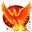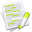#wx.lib.floatcanvas.FCObjects.XYObjectMixin¶

This is a mixin class that provides some methods suitable for use with objects that have a single (x,y) coordinate pair.

##Class Hierarchy¶Inheritance diagram for class XYObjectMixin:

##Methods Summary¶

 CalcBoundingBox Calculate the bounding box. Move Moves the object by delta, where delta is a (dx, dy) pair. SetPoint

##Class API¶

class XYObjectMixin

This is a mixin class that provides some methods suitable for use with objects that have a single (x,y) coordinate pair.

### Methods¶

CalcBoundingBox(self)

Calculate the bounding box.

Move(self, Delta)

Moves the object by delta, where delta is a (dx, dy) pair.

Parameters

Delta – is a (dx, dy) pair ideally a NumPy array of shape (2, )

SetPoint(self, xy)